Successfully reported this slideshow.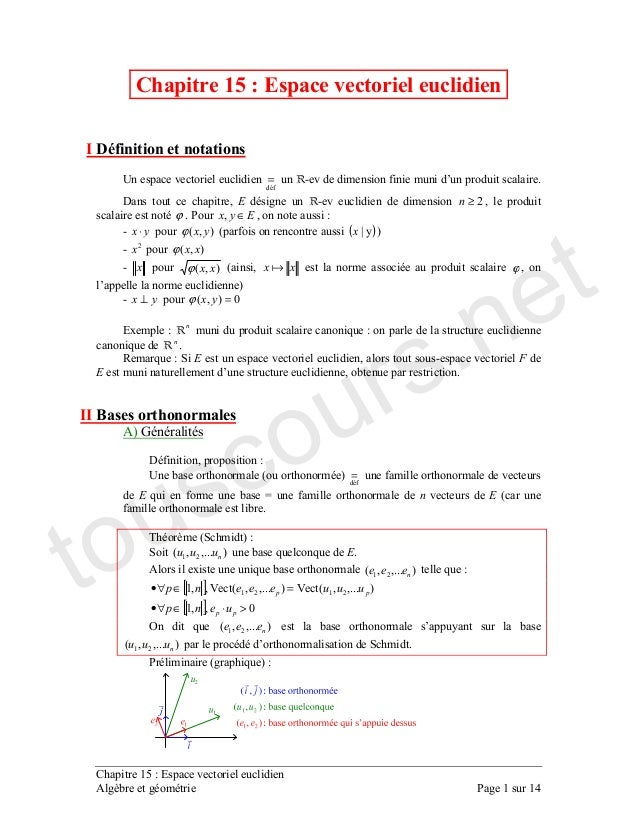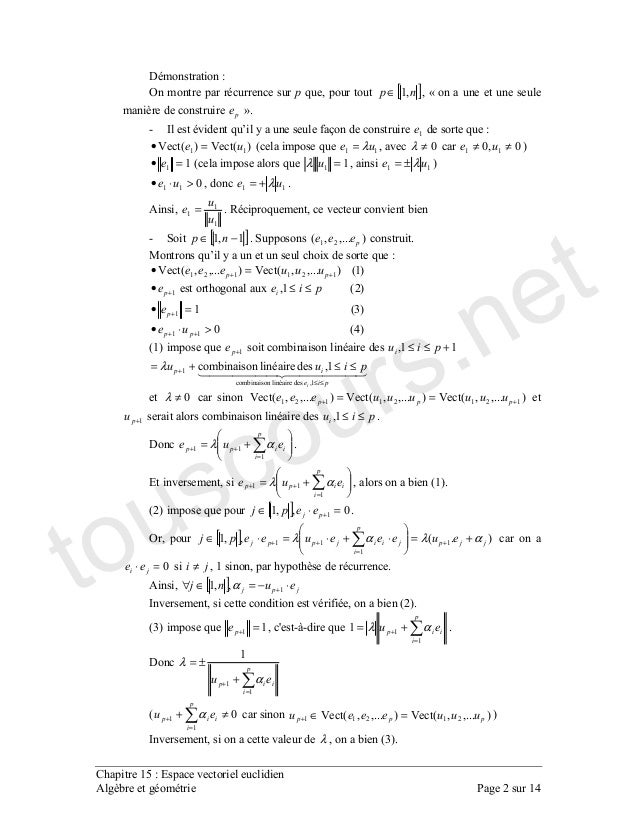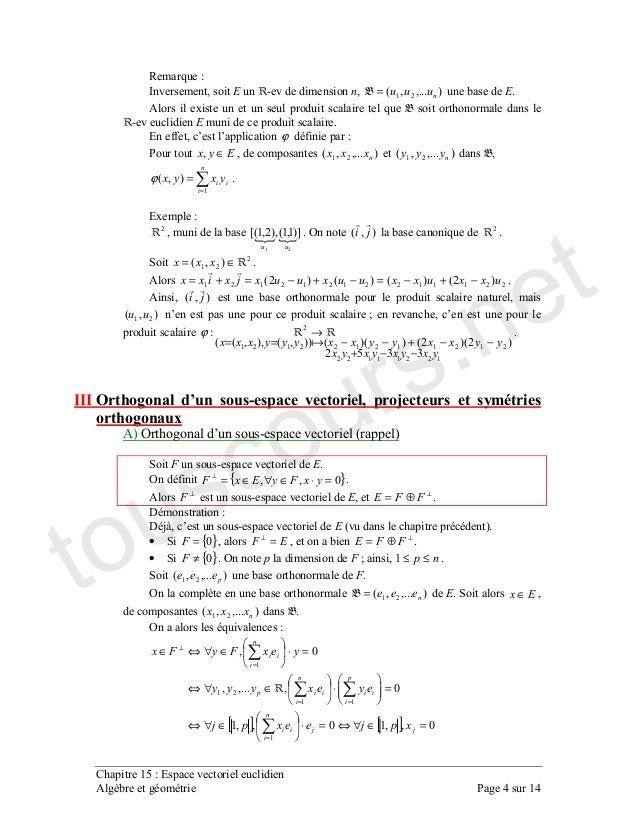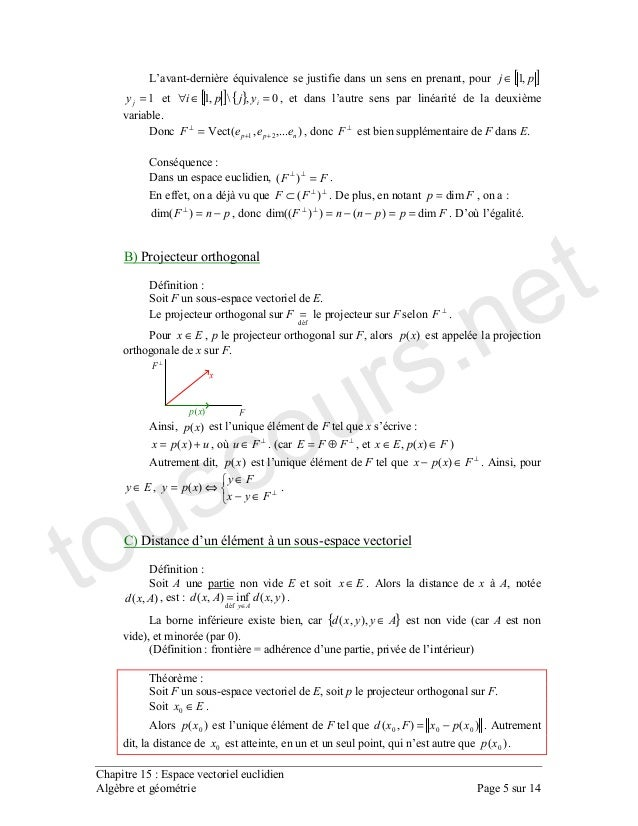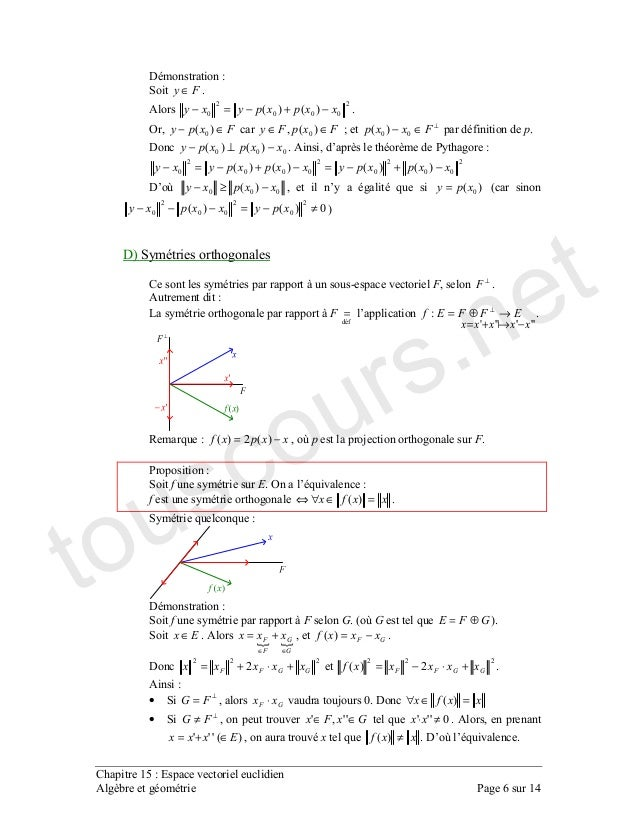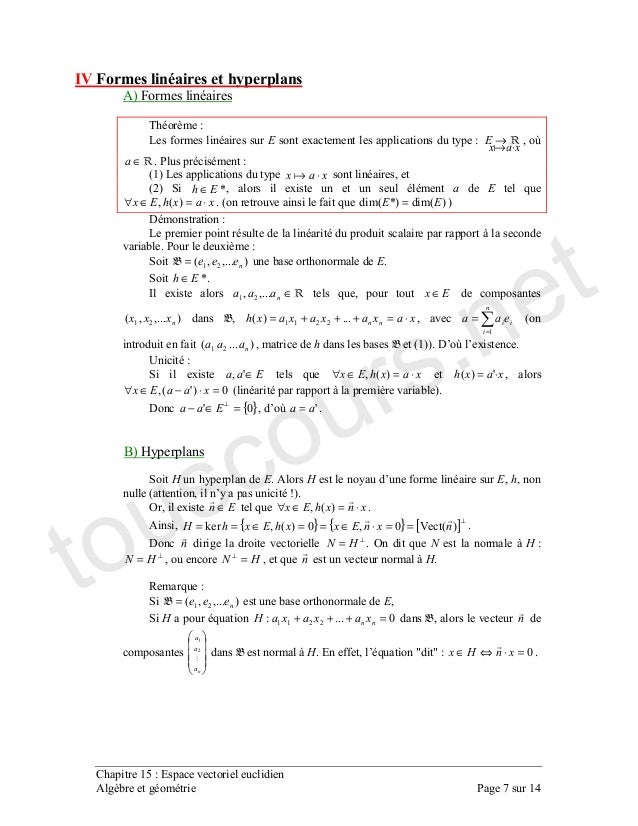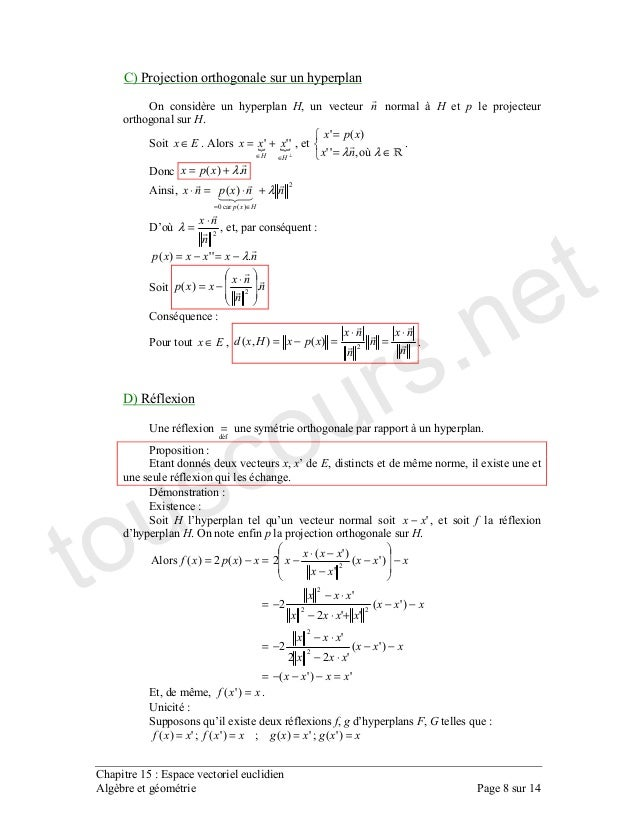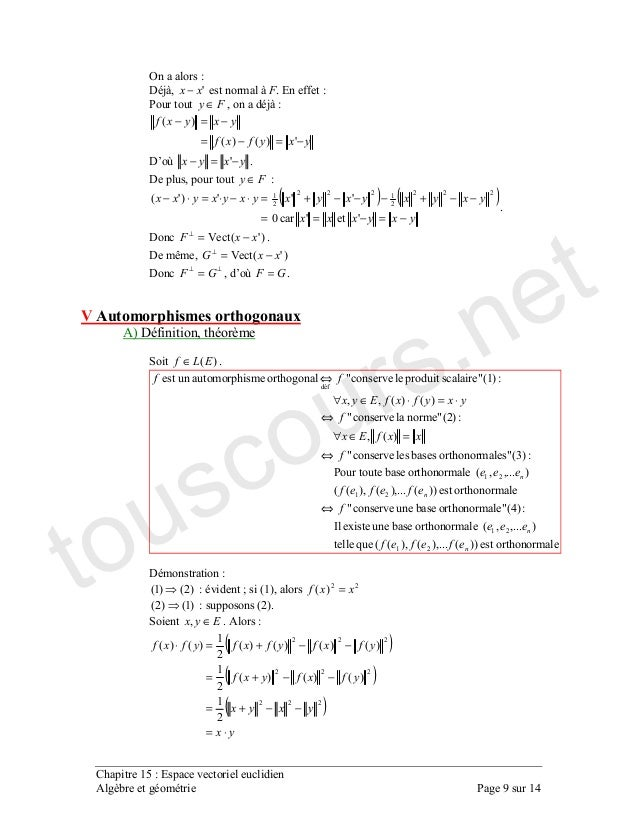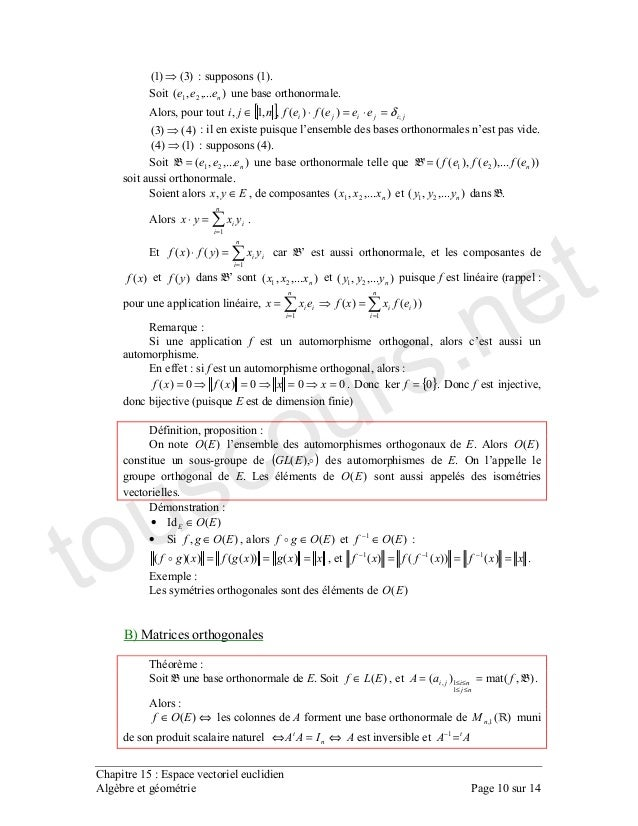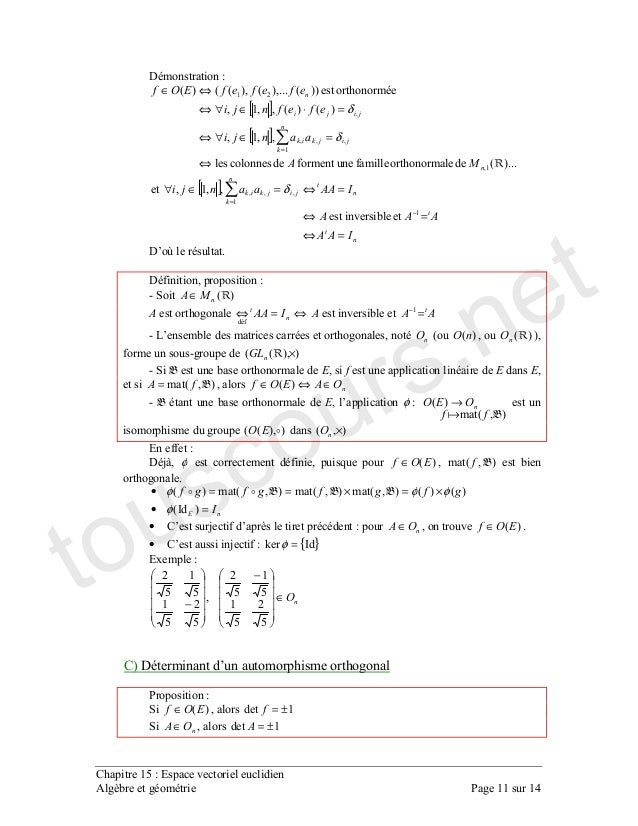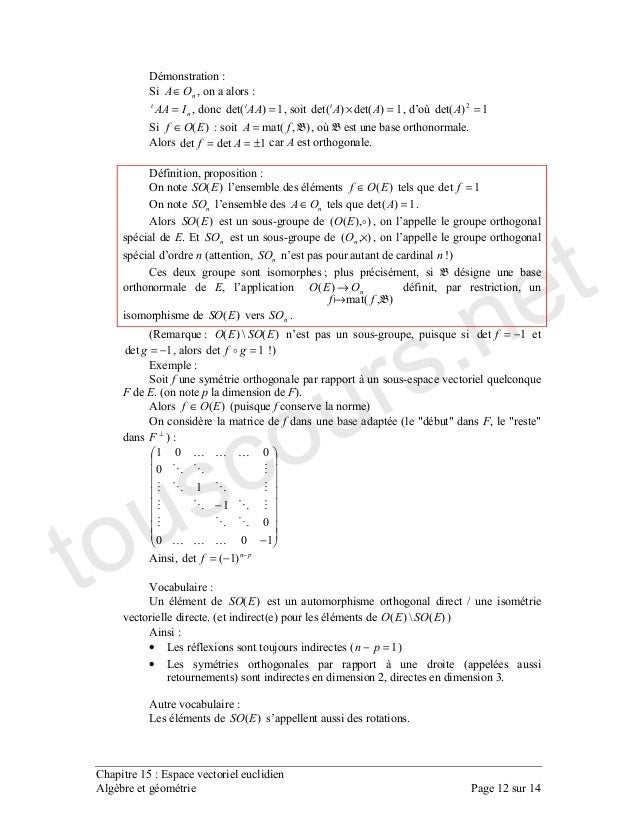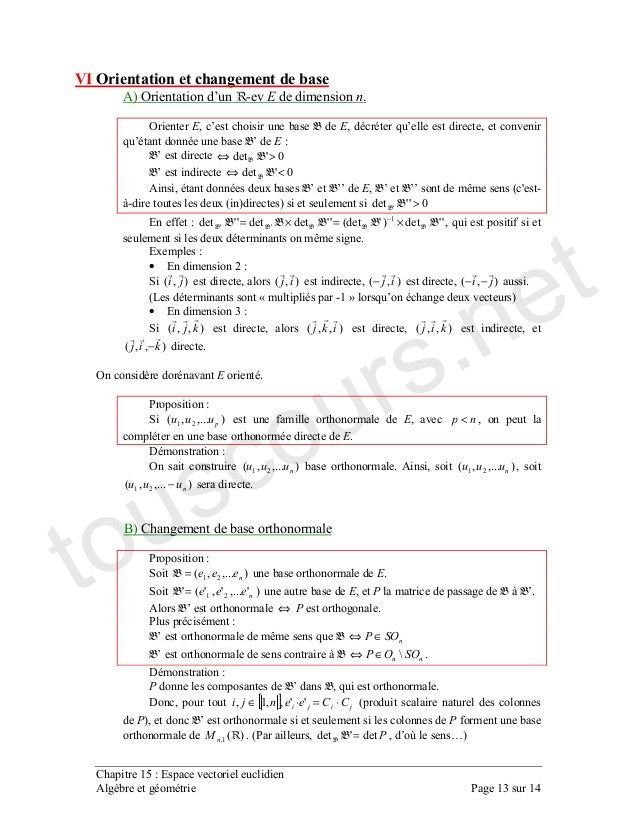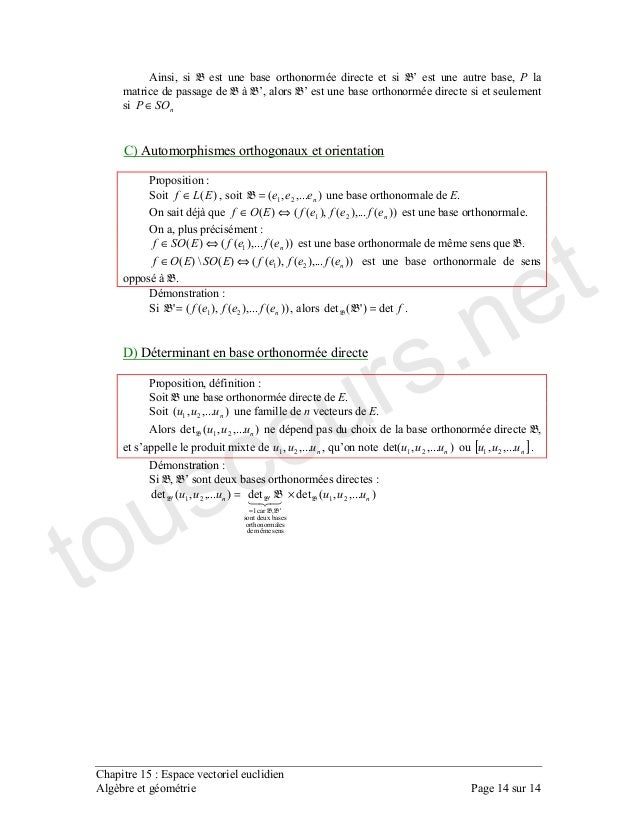Upcoming SlideShare
×
• Full Name
Comment goes here.

Are you sure you want to Yes No• Be the first to comment

• Be the first to like this

### Espace vectoriel euclidien

1. 1. = ! " # \$ %≥ \$ ϕ " ∈\$ \$ ⋅ &\$'ϕ ' ( )() & % &\$'ϕ &\$'ϕ ' \$ ϕ \$ ! & ⊥ *&\$' =ϕ + , , " - , . \$ ! \$ " & / # \$ ' & = , 0 ' " 1 '. & . &\$"""\$' % , , " + , &\$"""\$' % , [ ] [ ] *\$\$ &\$"""\$'2&\$"""\$'2\$\$ %% >⋅∈∀• =∈∀• 3 , &\$"""\$' % ! ( &\$"""\$' % ! . " ' , & touscours.net
2. 2. % # 3 , \$ [ ]\$∈ \$ 4 5" 6 , ! ( 7 , &'2&'2 =• ' , λ= \$ *≠λ *\$* ≠≠ & =• ' , =λ \$ λ±= & *>⋅• \$ λ+= " \$ = " - , \$ . [ ]\$ −∈ " . &\$"""\$' % " 8 , ! ( + , &'&\$"""\$'2&\$"""\$'2 %% ++ =• +• + &%'\$ ≤≤ &9'=• + &'*>⋅• ++ ' & , + \$ +≤≤ ≤≤ + ≤≤+= \$ \$λ *≠λ &\$"""\$'2&\$"""\$'2&\$"""\$'2 %%% ++ == + ≤≤\$ " # += = ++ αλ " \$ += = ++ αλ \$ ' &" '%& , [ ] *\$\$ =⋅∈ + " 3 \$ [ ] &"'\$\$ αλαλ +=⋅+⋅=⋅∈ + = ++ *=⋅ ≠ \$ \$ ( " \$ [ ] ⋅−=∈∀ +\$\$ α 6 \$ \$ '%&" '9& , =+ \$ : ; , = + += αλ " # = + + ±= α λ ' *≠+ = + α &\$"""\$'2&\$"""\$'2 %% =∈+ & 6 \$ λ \$ '9&" touscours.net
3. 3. 9 ' & + <\$ ⋅+⋅=⋅ = +++++ αλ " 3 \$ *=⋅ = +α + + ≤≤\$ " # *> ++ = ++ ⋅=⋅ λ *>λ " 6 \$ *>λ \$ ' &" , " , ' & # \$ + '%& 1 = " . &\$"""\$' % " \$ &\$"""\$\$"""\$' % + " ! . \$ &:\$""":\$:' % " 8 \$ ! . , &\$"""\$'2 %= \$ &:\$""":'&\$"""' = " >& . &\$"""\$' %= " . ∈ \$ &\$"""\$' % \$ = % " . ∈ \$ &\$"""\$' % \$ = % " 3 &'\$ [ ] &'' % % & \$\$ =×==⋅=⋅=⋅ =∈== \$ &'==⋅ = " \$ &'%% ==⋅= = \$ ! = → % &\$"""\$' φ \$ , \$ ?\$ , " '? : ; , ⋅=⋅∈ &'&'\$\$ φφ \$ &" touscours.net
4. 4. - , 6 \$ \$ &\$"""\$' %= " + , " \$ ! ! ϕ ∈\$ \$ &\$"""\$' % &\$"""\$' % \$ = =&\$'ϕ " + % \$ @&\$'\$&%\$'A % " 3 &\$' , % " . % % &\$' ∈= " %%%%%%% &%'&'&'&%' −+−=−+−=+= " \$ &\$' \$ &\$' % ! B \$ ! %%%% %%%%%% % 99% &%&'%'&&''&&\$'&\$\$'' −−+ −−+−−== →ϕ " ! " # & 3 ! ' & . " 3 { }*\$\$ =⋅∈∀∈=⊥ " ⊥ \$ ⊥ ⊕= " # # C;\$ ! ' &" • . { }*= \$ =⊥ \$ ⊥ ⊕= " • . { }*≠ " 3 B \$ ≤≤ " . &\$"""\$' % " 3 &\$"""\$' %= " . ∈ \$ &\$"""\$' % " 3 , [ ] [ ] *\$\$*\$\$ *\$\$"""\$ *\$ % =∈∀⇔=⋅∈∀⇔ =⋅∈∀⇔ =⋅∈∀⇔∈ = == = ⊥ touscours.net
5. 5. D! , C \$ [ ]\$∈ = [ ] { } *\$E\$ =∈∀ \$ ! + " # &\$"""\$'2 %++ ⊥ = \$ ⊥ " , # \$ =⊥⊥ &' " \$ C; , ⊥⊥ ⊂ &' " # \$ = \$ −=⊥ &' \$ &'&&'' ==−−=⊥⊥ " #!F ! " >& C # . " D C = C ⊥ " ∈ \$ C \$ &' C " ⊥ \$ &' ! , , ! += &' \$ F ⊥ ∈ " ' ⊥ ⊕= \$ ∈∈ &'\$ & \$ &' ! , , ⊥ ∈− &' " \$ ∈ \$ ∈− ∈ ⇔= ⊥ &' " & # ! ; # . ∈ " ; \$ &\$' \$ &\$'&\$' ∈ = " D + \$ { }∈&\$\$' ' &\$ ' *&" '# 0 ! \$ ! & 1 . \$ C " . ∈* " &' * ! , , &'&\$' *** −= " \$ * \$ \$ , ! , &' * " touscours.net
6. 6. G # . ∈ " % *** % * &'&' −+−=− " 3 \$ ∈− &' * ∈∈ &'\$ * B ⊥ ∈− ** &' " # *** &'&' −⊥− " \$ ! ( % ** % * % *** % * &'&'&'&' −+−=−+−=− #!F *** &' −≥− \$ !( , &' *= ' *&'&' % * % ** % * ≠−=−−− & #& .( ( ; \$ ⊥ " D ( ; = ! :::::: −+= →⊕= ⊥ " ⊥ - , −= &'%&' \$ F C " . ( " 3 !, ( =∈∀⇔ &' " .( , , # . ( ; " ' F , ⊕= &" . ∈ " ∈∈ += \$ −=&' " # %%% % +⋅+= %%% %&' +⋅−= " • . ⊥ = \$ ⋅ C *" # =∈∀ &' • . ⊥ ≠ \$ ∈∈ ::\$: , *::: ≠⋅ " \$ &'::: ∈+= \$ , ≠&' " #!F !, " touscours.net
7. 7. H \$ % " & I 1 D + ( ⋅ → \$ F ∈ " ' & D ( ⋅ \$ '%& . ?∈ \$ + , ⋅=∈∀ &'\$ " ' , &'?&' = & # D ; " + . &\$"""\$' %= " . ?∈ " 6 + ∈\$"""\$ % , \$ ∈ &\$"""\$' % \$ ⋅=+++= """&' %% \$ = = ' &"""' % \$ ' &&" #!F !+ " . + ∈:\$ , ⋅=∈∀ &'\$ ⋅= :&' \$ *&:'\$ =⋅−∈∀ ' ; &" # { }*: =∈− ⊥ \$ !F := " >& J( . ( " ( ! \$ \$ ' \$ !( K&" 3 \$ + ∈ , ⋅=∈∀ &'\$ " \$ { } { } [ ]⊥ ==⋅∈==∈== &'2*\$*&'\$L " # ⊥ = " 3 , ; ⊥ = \$ =⊥ \$ , ; " - , . &\$"""\$' %= \$ . , *"""%% =+++ \$ % ; " \$ !, M M *=⋅⇔∈ " touscours.net
8. 8. N & C ( 3 ( \$ ; C " . ∈ " ⊥ ∈∈ += ::: \$ ∈= = λλ F\$:: &': " # "&' λ+= \$ % &'* &' λ+⋅=⋅ ∈= #!F % ⋅ =λ \$ \$ , "::&' λ−=−= . "&' % ⋅ −= , ∈ \$ ⋅ = ⋅ =−= % &'&\$' " #& - + + = ( ; ( " + \$ ! \$ = \$ + + , " # + . !( , ! :− \$ + !( " 3 C " :&:' &:' :%% : % &:' ::% : % &:' : &:' %&'%&' % % %% % % =−−−= −− ⋅− ⋅− −= −− +⋅− ⋅− −= −− − −⋅ −=−= \$ = \$ =&:' " . , ! + + + \$ !( \$ , ==== &:'B:&'B&:'B:&' touscours.net
9. 9. O 3 # C;\$ :− ; " ∈ \$ C; −=−= −=− :&'&' &' #!F −=− : " # \$ ∈ ( ) ( ) −=−== −−+−−−+=⋅−⋅=⋅− ::* :::&:' %%% % %%% % " # &:'2 −=⊥ " # = \$ &:'2 −=⊥ # ⊥⊥ = \$ !F = " \$ & # & # \$ . &'∈ " &&'&\$"""'&\$'', &\$"""\$'+6 ' &MM &&'&\$"""'&\$'' &\$"""\$' '9&MM &'\$ '%&MM &'&'\$\$ ' &MM % % % % ⇔ ⇔ =∈∀ ⇔ ⋅=⋅∈∀ ⇔ # &%'&' B ' &\$ %% &' = &'&%' '%&" . ∈\$ " ( ) ( ) ( ) ⋅= −−+= −−+= −−+=⋅ %%% %%% %%% % &'&'&' % &'&'&'&' % &'&' touscours.net
10. 10. * &9'&' ' &" . &\$"""\$' % " \$ [ ] B&'&'\$\$\$ δ=⋅=⋅∈ &'&9' + , ! ! " &'&' ' &" . &\$"""\$' %= , &&'&\$"""'&\$'': %= " . ∈\$ \$ &\$"""\$' % &\$"""\$' % " = =⋅ " = =⋅ &'&' ! \$ &' &' ! &\$"""\$' % &\$"""\$' % , ' \$ == == &'&' & - , . \$ ! " \$ ***&'*&' ==== " # { }*L = " # C \$ C ' , & # \$ 3 &' ! + " &' ( )&\$' " 3 ! " D &' " # &'6 ∈• • . &'\$ ∈ \$ &'∈ &'∈− === &'&&''&&'' \$ === −−− &'&&''&' " + D ( &' >& 8 1 . " . &'∈ \$ &\$'&' \$ == ≤≤ ≤≤ " ⇔∈ &' &'\$ ⇔=⇔ =− touscours.net
11. 11. # [ ] [ ] &"""' \$\$\$ &'&'\$\$\$ &&'&\$"""'&\$''&' \$ \$\$\$ \$ % ⇔ =∈∀⇔ =⋅∈∀⇔ ⇔∈ = δ δ [ ] =⇔ =⇔ =⇔=∈∀ − = \$\$\$\$\$\$ δ #!F " # \$ . &'∈ ⇔=⇔ =− D! \$ ' &' \$ &' &\$ &&\$'' × . \$ \$ &\$'= \$ ∈⇔∈ &' \$ ! &\$' &' →φ &&\$'' &\$' × # C;\$ φ \$ , &'∈ \$ &\$' " =• ×=×==• &6' &'&'&\$'&\$'&\$'&' φ φφφ • ! C ! ∈ \$ &'∈ " • ! C { }6L =φ + ∈ − − % % \$ % % & # ! . &'∈ \$ ±= . ∈ \$ ±= touscours.net
12. 12. % # . ∈ \$ = \$ &' = \$ &'&' =× \$ !F &' % = . &'∈ &\$'= \$ F " ±== " # \$ 3 &' ! &'∈ , = 3 ! ∈ , &' = " &' &&\$'' \$ ! " &\$' × \$ ! ! ' \$ ! K& + B \$ \$ ! &\$' &' → \$ \$ &' " '- , &'E&' ! \$ , −= −= \$ = K& + . ( ; , , " ' &" &'∈ ' , & 3 ' M M \$ M M ⊥ & − − ** * * ** \$ − −= &' 2 &' P " ' ' & &'E&' & • D + C ' =− & • D ( ; ' & %\$ 9" D &' ! " touscours.net
13. 13. 9 \$ ' & 3 ! " 3 \$ ! \$ , ! \$ , ! ! ! *:>⇔ ! *:<⇔ \$ + ! !! \$ ! !! = ' : ; + ' & & *::: > ::&:':::: :: ×=×= − \$ , + = " + • % . &\$' \$ &\$' \$ &\$'− \$ &\$' −− " 'D 4 5 , ! + & • 9 . &\$\$' \$ &\$\$' \$ &\$\$' \$ &\$\$' − " 3 " . &\$"""\$' % \$ < \$ " # 3 &\$"""\$' % " \$ &\$"""\$' % \$ &\$"""\$' % − " >& . &\$"""\$' %= " . &:\$""":\$:': %= \$ ; !" ! ⇔ " ! = , ∈⇔ ! ; E∈⇔ " # ! \$ , " # \$ [ ] ⋅=⋅∈ ::\$\$\$ ' &\$ ! &'\$ " ' \$ := \$ !F Q & touscours.net
14. 14. \$ ! \$ ; !\$ ! ∈ & + . &'∈ \$ &\$"""\$' %= " 3 C; , &&'&\$"""'&\$''&' %⇔∈ " 3 \$ &&'&\$"""''&' ⇔∈ = , " &&'&\$"""'&\$''&'E&' %⇔∈ ; " # . &&'&\$"""'&\$'': %= \$ &:' = " #& # \$ . " . &\$"""\$' % " &\$"""\$' % + \$ ! + \$"""\$ % \$ , ! &\$"""\$' % [ ]\$"""\$ % " # . \$ ! + &\$"""\$'&\$"""\$' % = + :\$ :%: ×= = touscours.net